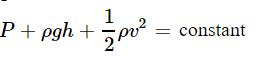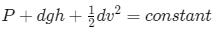Courses

# Test: Applications of Bernoulli’s Principle

## 10 Questions MCQ Test Physics For JEE | Test: Applications of Bernoulli’s Principle

Description
This mock test of Test: Applications of Bernoulli’s Principle for JEE helps you for every JEE entrance exam. This contains 10 Multiple Choice Questions for JEE Test: Applications of Bernoulli’s Principle (mcq) to study with solutions a complete question bank. The solved questions answers in this Test: Applications of Bernoulli’s Principle quiz give you a good mix of easy questions and tough questions. JEE students definitely take this Test: Applications of Bernoulli’s Principle exercise for a better result in the exam. You can find other Test: Applications of Bernoulli’s Principle extra questions, long questions & short questions for JEE on EduRev as well by searching above.
QUESTION: 1

### Bernoulli’s theorem includes as a special case of:

Solution:

Torricelli's law states that the speed of efflux, v of a fluid through a sharp-edged hole at the bottom of a tank filled to a depth h is the same as the speed that a body (in this case a drop of water) would acquire in falling freely from a height h.
This problem is solved using Bernoulli's equation,
gh+Patm/ρ​​=v2/2​+Patm
⟹v=√2gh​

QUESTION: 2

### Bernoulli’s theorem is important in the field of:

Solution:

The Bernoulli equation is an important expression relating pressure, height and velocity of a fluid at one point along its flow.

QUESTION: 3

### Water is flowing through a horizontal tube. The pressure of the liquid in the portion where velocity is 2 m/s is 2 m of Hg. What will be the pressure in the portion where velocity is 4 m/s?

Solution:QUESTION: 4

Air is streaming past a horizontal airplane wing such that its speed is 120 m/s over the upper surface and 90 m/s at the lower surface. If the density of the air is 1.3 kg/m3and the wing is 10 m long and has an average width of 2 m, then the difference of pressure on the two sides of the wing is

Solution:

Applying Bernoulli's principle, we have
P1+1/2​ρv12=P2+1/2​ρv22
⇒P2​−P1=1/2ρ (v2/1−v2/2)
⇒ΔP=1/2​×1.3× (1202−902)
⇒ΔP=0.65× (120+90) × (120−90)
⇒ΔP=0.65×210×30=4095Pa

QUESTION: 5

In which of the following types of flows is the Bernoulli’s theorem strictly applicable:

Solution:

Bernoulli's principle is applicable on those non-viscous liquids which have laminar flow or streamlined flow.
It means that a liquid in which its particles exert no force on each other, i.e., the speed of all the particles of the liquid is same.
Also, the liquid should travel in the form of streamlines, i.e., the liquid flowing in one pipeline (imaginary pipeline) should not mix in the liquid in another pipeline. This is called streamlined flow.
Also, turbulent flow is the opposite of streamlined flow. So, turbulent liquid will not obey Bernoulli's principle.
But if the liquid is rotated, this streamlined flow will not take place. Thus Bernoulli's principle will not be applicable to this type of liquid.

QUESTION: 6

In the houses far away from the municipal water tanks often people find it difficult to get water on the top floor. This happens because

Solution:

In the houses far away from the municipal water tanks often people find it difficult to get water on the top floor. This happens because a lot of water pressure is lost as friction between water and the pipeline.

QUESTION: 7

Water is flowing through a pipe under constant pressure. At some place the pipe becomes narrow. The pressure of water at this place:

Solution:

Given, That area of cross section at initial point is more than the final point,

From the equation of continuity, we can say that velocity at the final point is more than initial point.

Bernoulli theorem states thatAt final point, the velocity is more, so the pressure will be less.

Therefore the pressure decreases

QUESTION: 8

Water is flowing through a horizontal pipe in streamline flow at the narrowest part of the pipe:

Solution:

Since Av has to be constant so as A decreases v has to increase and according to Bernaulli’s Theorem the sum of energies should be conserved. Since KE is high the pressure energy should be low.

QUESTION: 9

To which type of fluid is the Bernoulli’s theorem applicable:

Solution:

Bernoulli's theorem, in fluid dynamics, relation among the pressure, velocity, and elevation in a moving fluid (liquid or gas), the compressibility and viscosity (internal friction) of which are negligible and the flow of which is steady, or laminar.
Bernoulli's principle is applicable on those non-viscous liquids which have laminar flow or streamlined flow.
It means that a liquid in which its particles exert no force on each other, i.e., the speed of all the particles of the liquid is same.
Also, the liquid should travel in the form of streamlines, i.e., the liquid flowing in one pipeline (imaginary pipeline) should not mix in the liquid in another pipeline. This is called streamlined flow.
Also, turbulent flow is the opposite of streamlined flow. So, turbulent liquid will not obey Bernoulli's principle.
But if the liquid is rotated, this streamlined flow will not take place. Thus Bernoulli's principle will not be applicable to this type of liquid.

QUESTION: 10

Bernoulli’s principle is based on the conservation of:

Solution:

Bernoulli's principle can even be derived from the principle of conservation of energy. Bernoulli's principle states that, in a steady flow, the sum of all forms of energy in a fluid along a streamline is the same at all points on that streamline.